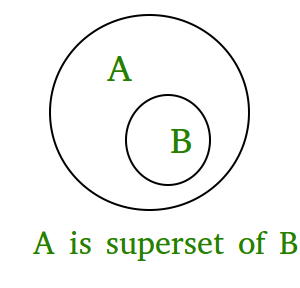# issuperset() in Python

The issuperset() method returns True if all elements of a set A occupies set B which is passed as an argument and returns false if all elements of B not present in A.
This means if A is a superset of B then it returns true; else False

Syntax:

```A.issuperset(B)
checks whether A is a superset of B or not.```

Returns:

`True if A is a superset of B; otherwise false.`Code 1:

 `# Python program to demonstrate working of ` `# issuperset(). ` ` `  `A ``=` `{``4``, ``1``, ``3``, ``5``} ` `B ``=` `{``6``, ``0``, ``4``, ``1``, ``5``, ``0``, ``3``, ``5``} ` ` `  `print``(``"A.issuperset(B) : "``, A.issuperset(B)) ` ` `  `# B is superset of A ` `print``(``"B.issuperset(A) : "``, B.issuperset(A)) `

Output:

```A.issuperset(B) :  False
B.issuperset(A) :  True
```

Code 2:

 `# Python program to demonstrate working  ` `# of issuperset(). ` ` `  `A ``=` `{``1``, ``2``, ``3``} ` `B ``=` `{``1``, ``2``, ``3``, ``4``, ``5``} ` `C ``=` `{``1``, ``2``, ``4``, ``5``} ` ` `  `print``(``"A.issuperset(B) : "``, A.issuperset(B)) ` ` `  `print``(``"B.issuperset(A) : "``, B.issuperset(A)) ` ` `  `print``(``"A.issuperset(C) : "``, A.issuperset(C)) ` ` `  `print``(``"C.issuperset(B) : "``, C.issuperset(B)) `

Output:

```A.issuperset(B) :  False
B.issuperset(A) :  True
A.issuperset(C) :  False
C.issuperset(B) :  False```

My Personal Notes arrow_drop_upIf you like GeeksforGeeks and would like to contribute, you can also write an article using contribute.geeksforgeeks.org or mail your article to contribute@geeksforgeeks.org. See your article appearing on the GeeksforGeeks main page and help other Geeks.

Please Improve this article if you find anything incorrect by clicking on the "Improve Article" button below.

Article Tags :
Practice Tags :

3

Please write to us at contribute@geeksforgeeks.org to report any issue with the above content.